# Unicus Olympiads - UCTO PDF Sample Papers for Class 10

Class 10 sample paper & practice questions for Unicus Critical Thinking Olympiad (UCTO) PDF Sample Papers are given below. Syllabus for UCTO is also mentioned for these exams. You can refer these sample paper & quiz for preparing for the UCTO exam.#### Resources:

##### Sample Questions from Olympiad Success:
 Q.1 Q.2 Q.3 Q.4 Q.5 Q.6 Q.7 Q.8 Q.9 Q.10
 Q.1 If in a certain code language, 'LAP' is coded as 'KMZBOQ', then how will 'NOTE' be written in that language? a) MONPSUFD b) MONPUSDF c) MNOPSUDF d) MONPSUDF
 Q.2 A rat runs 20 m towards East and turns to the right, runs 10 m and turns to the right, runs 9 m and again turns to left, runs 5 m and then turns to left, runs 12 m and finally turns to left and runs 6 m. Now, in which direction is the rat facing? a) South b) West c) East d) North
 Q.3 Two positions of a dice are given below. If there's one dot at the top, how many dots will be there at the bottom?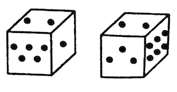a) 2 b) 3 c) 4 d) 6
 Q.4 Answer the question based on the following arrangement:M 7 Σ 8 L P @ ? 6 N B T Y 3 2 = E \$ 4 9 © G H 5 If all the symbols are deleted from the arrangement, then will which of the following will be fourth to the left of the 17th element from the left end? a) 9 b) 2 c) E d) Y
 Q.5 B is the father of Q. B has only two children. Q is the brother of R. R is the daughter of P. A is the granddaughter of P and S is the father of A. How is S related to Q? a) Son b) Son-in-law c) Brother d) Brother-in-law
 Q.6 Four pairs of coloured socks, namely. black, white, green and orange are mixed in a box. Each of the four blind friends A, B, C and D picks up a pair of socks from the box. None of them gets the right pair. A and C do not get any common colour. Likewise, B and D also have no colour in common. The pairs of A and B give a pair of white coloured socks. A doesn't have any orange coloured socks and C doesn't have any green coloured socks. A pair of green coloured socks can be obtained from the pairs of? a) A and D b) B and C c) B and D d) C and D
 Q.7 Select the option figure which satisfies the same conditions of the placement of dots as in the question figure: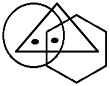a)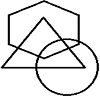b)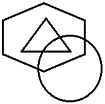c)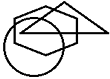d)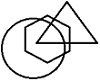Q.8 Use the relationships defined below to solve the question:(i) 'P × Q' means 'P is the father of Q'.(ii) 'P * Q' means 'P is the sister of Q'.(iii) 'P + Q' means 'P is the brother of Q'.(iv) 'P - Q' means 'P is the mother of Q'.(v) 'P ÷ Q' means 'P is the son of Q'.(vi) 'P = Q' means 'P is the daughter of Q'. Which of the following means Z is the brother of X? a) Y ÷ Z - X b) Z ÷ Y - X c) Y = X - Z d) X ÷ Y × Z
 Q.9 Read the following information carefully to answer the question that follows:Nine professors - G, H, I, J, K, L, M, N and O are appear on a series of three panels. Each panel will consists of three professors and each professor will appear exactly once. The panel must be arranged according to the following conditions.I. I and N must be on the same panel.II. K and L must be on the same panel.III. O and J cannot be on the same panel.IV. M must appear on the second panel.V. Either J or M or both must appear on the panel with H. If J and K appear on the 3rd panel, which of the following professors must appear on 2nd? a) G b) H c) I d) L
 Q.10 In the following question, a sheet of paper is folded and punch is made. When unfolded the paper sheet looks like the question figure. See the answer figures and select the one that follows the manner in which the paper is folded and punch is made: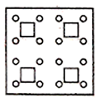a)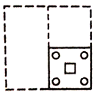b)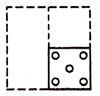c)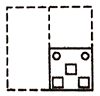d)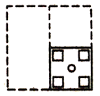Sample PDF of Unicus Olympiads - Unicus Critical Thinking Olympiad (UCTO) PDF Sample Papers for Class 10:

 Q.1 )d Q.2 )d Q.3 )d Q.4 )c Q.5 )d Q.6 )a Q.7 )a Q.8 )b Q.9 )b Q.10 )a

Q.1 : d | Q.2 : d | Q.3 : d | Q.4 : c | Q.5 : d | Q.6 : a | Q.7 : a | Q.8 : b | Q.9 : b | Q.10 : a